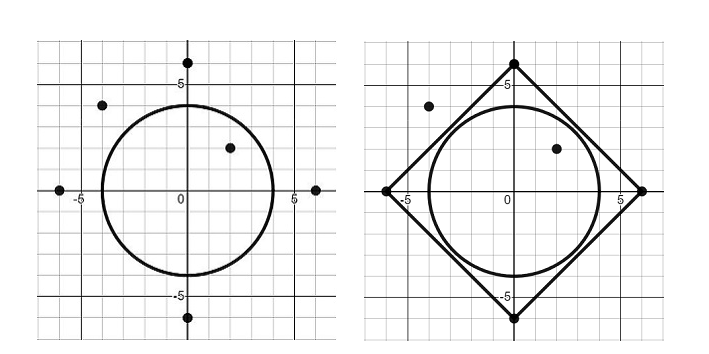# Cold Rainy Night in StokeIftekhar_HakimBUET Inter University Pro...3/4/41
Limits 1s, 256 MB

Night is ending. You have just got $N$ points in 2D-coordinate plane in your dream. Wait! Things were going well with the points. Suddenly, a circle appeared, which is centered at coordinate $(0,0)$ and has a radius of $R$. Your mind is telling you to create a convex polygon, whose vertices are subset of those $N$ points. This convex polygon must enclose the circle completely. That is, no point of the circle (including the points on its boundary) is strictly outside the polygon. The polygon may touch the circle.

This is not enough. You have to find the polygon with smallest area. Output the value of the smallest area$\times 2$. If it is impossible to construct such a polygon, output should be $-1$.

Let’s just solve this problem before the night ends.

## Input

First line of the input will have an integer $T$ $(1 \le T \le 100)$, denoting the number of testcases. Each testcase is independent.

First line of each testcase will have an integer $N$ $(1 \le N \le 500)$, denoting the number of points you have got.

Line $i$ of the next $N$ lines will have two integers $x_i$ and $y_i$ $(-10^4 \le x_i, y_i \le 10^4)$ denoting the abscissa and ordinate $i$-th point respectively, for $i=1, 2, \dots, N$.

Last line of each testcase will have an integer $R$ $(1\le R \le 10^4)$, denoting the radius of the circle.

Sum of $N$ over all testcases will not exceed $500$.

## Output

Print a separate line for each testcase. Line $i$ of the output should have the output for $i$-th testcase. Output of the $i$-th testcase is the value of $2 \times A$, where $A$ is the area of the smallest possible convex polygon. If there is no possible convex polygon, output is $-1$.

## Sample

InputOutput
2
6
-6 0
-4 4
0 6
0 -6
2 2
6 0
4
1
5 5
1

144
-1


Following figures show the input of 1st testcase and the convex polygon with smallest area. Here the area is $72$.### Submit